xAirspeedEncyclopedia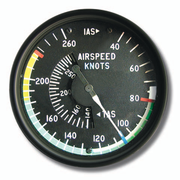Airspeed is the speed of an aircraft
Aircraft
An aircraft is a vehicle that is able to fly by gaining support from the air, or, in general, the atmosphere of a planet. An aircraft counters the force of gravity by using either static lift or by using the dynamic lift of an airfoil, or in a few cases the downward thrust from jet engines.Although...

relative to the air. Among the common conventions for qualifying airspeed are: indicated airspeed ("IAS"), calibrated airspeed ("CAS"), true airspeed ("TAS"), equivalent airspeed ("EAS") and density airspeed.

The measurement and indication of airspeed is ordinarily accomplished on board an aircraft by an airspeed indicator
Airspeed indicator
The airspeed indicator or airspeed gauge is an instrument used in an aircraft to display the craft's airspeed, typically in knots, to the pilot.- Use :...

("ASI") connected to a pitot-static system. The pitot-static system comprises one or more pitot probes
Pitot tube
A pitot tube is a pressure measurement instrument used to measure fluid flow velocity. The pitot tube was invented by the French engineer Henri Pitot Ulo in the early 18th century and was modified to its modern form in the mid-19th century by French scientist Henry Darcy...

(or tubes) facing the on-coming air flow to measure pitot pressure (also called stagnation
Stagnation pressure
In fluid dynamics, stagnation pressure is the static pressure at a stagnation point in a fluid flow.At a stagnation point the fluid velocity is zero and all kinetic energy has been converted into pressure energy . Stagnation pressure is equal to the sum of the free-stream dynamic pressure and...

, total or ram pressure) and one or more static ports to measure the static pressure in the air flow. These two pressures are compared by the ASI to give an IAS reading.

## Indicated airspeed

Indicated airspeed
Indicated airspeed
Indicated airspeed is the airspeed read directly from the airspeed indicator on an aircraft, driven by the pitot-static system. IAS is directly related to calibrated airspeed , which is the IAS corrected for instrument and installation errors....

(IAS) is the airspeed indicator
Airspeed indicator
The airspeed indicator or airspeed gauge is an instrument used in an aircraft to display the craft's airspeed, typically in knots, to the pilot.- Use :...

reading (ASIR) uncorrected for instrument, position, and other errors. From current EASA definitions: Indicated airspeed means the speed of an aircraft as shown on its pitot static airspeed indicator calibrated to reflect standard atmosphere adiabatic compressible flow at sea level uncorrected for airspeed system errors.

Outside of the former Soviet bloc, most airspeed indicators show the speed in knots i.e. nautical miles per hour. Some light aircraft have airspeed indicators showing speed in miles per hour.

An airspeed indicator
Airspeed indicator
The airspeed indicator or airspeed gauge is an instrument used in an aircraft to display the craft's airspeed, typically in knots, to the pilot.- Use :...

is a differential pressure gauge with the pressure reading expressed in units of speed, rather than pressure. The airspeed is derived from the difference between the ram air pressure from the pitot tube, or stagnation pressure
Stagnation pressure
In fluid dynamics, stagnation pressure is the static pressure at a stagnation point in a fluid flow.At a stagnation point the fluid velocity is zero and all kinetic energy has been converted into pressure energy . Stagnation pressure is equal to the sum of the free-stream dynamic pressure and...

, and the static pressure
Static pressure
In fluid mechanics the term static pressure has several uses:* In the design and operation of aircraft, static pressure is the air pressure in the aircraft’s static pressure system....

. The pitot tube is mounted facing forward; the static pressure is frequently detected at static ports on one or both sides of the aircraft. Sometimes both pressure sources are combined in a single probe, a pitot-static tube
Pitot tube
A pitot tube is a pressure measurement instrument used to measure fluid flow velocity. The pitot tube was invented by the French engineer Henri Pitot Ulo in the early 18th century and was modified to its modern form in the mid-19th century by French scientist Henry Darcy...

. The static pressure measurement is subject to error due to inability to place the static ports at positions where the pressure is true static pressure at all airspeeds and attitudes. The correction for this error is the position error
Position error
Position error is one of the errors affecting the systems in an aircraft for measuring airspeed and altitude. It is not practical or necessary for an aircraft to have an airspeed indicating system and an altitude indicating system that are exactly accurate...

correction (PEC) and varies for different aircraft and airspeeds. Further errors of 10% or more are common if the airplane is flown in “uncoordinated” flight.

## Calibrated airspeed

Calibrated airspeed
Calibrated airspeed
Calibrated airspeed is the speed shown by a conventional airspeed indicator after correction for instrument error and position error. Most civilian EFIS displays also show CAS...

(CAS) is indicated airspeed corrected for instrument errors, position error (due to incorrect pressure at the static port) and installation errors.

Calibrated airspeed values less than the speed of sound
Speed of sound
The speed of sound is the distance travelled during a unit of time by a sound wave propagating through an elastic medium. In dry air at , the speed of sound is . This is , or about one kilometer in three seconds or approximately one mile in five seconds....

at standard sea level (661.4788 knots) are calculated as follows:minus position and installation error correction.

Where :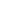is the calibrated airspeed,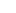is the impact pressure
Impact pressure
In compressible fluid dynamics, impact pressure is the difference between total pressure and static pressure. In aerodynamics notation, this quantity is denoted as q_c or Q_c....

(inches Hg) sensed by the pitot tube,is 29.92126 inches Hg; static air pressure at standard sea level,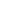is 661.4788 knots;, speed of sound at standard sea level.

Units other than knots and inches of mercury can be used, if used consistently.

This expression is based on the form of Bernoulli's equation applicable to a perfect, compressible gas. The values forand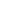are consistent with the ISA
International Standard Atmosphere
The International Standard Atmosphere is an atmospheric model of how the pressure, temperature, density, and viscosity of the Earth's atmosphere change over a wide range of altitudes. It has been established to provide a common reference for temperature and pressure and consists of tables of...

i.e. the conditions under which airspeed indicators are calibrated.

## Equivalent airspeed

Equivalent airspeed
Equivalent airspeed
Equivalent airspeed is the airspeed at sea level in the International Standard Atmosphere at which the dynamic pressure is the same as the dynamic pressure at the true airspeed and altitude at which the aircraft is flying. In low-speed flight, it is the speed which would be shown by an airspeed...

(EAS) is defined as the speed at sea level that would produce the same incompressible dynamic pressure as the true airspeed at the altitude at which the vehicle is flying. An aircraft in forward flight is subject to the effects of compressibility. Likewise, the calibrated airspeed is a function of the compressible impact pressure. EAS, on the other hand, is a measure of airspeed that is a function of incompressible dynamic pressure. Structural analysis is often in terms of incompressible dynamic pressure, so that equivalent airspeed is a useful speed for structural testing. At standard
International Standard Atmosphere
The International Standard Atmosphere is an atmospheric model of how the pressure, temperature, density, and viscosity of the Earth's atmosphere change over a wide range of altitudes. It has been established to provide a common reference for temperature and pressure and consists of tables of...

sea level pressure, calibrated airspeed and equivalent airspeed are equal. Up to about 200 knots CAS and 10,000 feet the difference is negligible, but at higher speeds and altitudes CAS must be corrected for compressibility error to determine EAS. The significance of equivalent airspeed is that at Mach numbers below the onset of wave drag, all of the aerodynamic forces and moments on an aircraft scale with the square of the equivalent airspeed. The equivalent airspeed is closely related to the indicated airspeed
Indicated airspeed
Indicated airspeed is the airspeed read directly from the airspeed indicator on an aircraft, driven by the pitot-static system. IAS is directly related to calibrated airspeed , which is the IAS corrected for instrument and installation errors....

shown by the airspeed indicator
Airspeed indicator
The airspeed indicator or airspeed gauge is an instrument used in an aircraft to display the craft's airspeed, typically in knots, to the pilot.- Use :...

. Thus, the handling and 'feel' of an aircraft, and the aerodynamic loads upon it, at a given equivalent airspeed, are very nearly constant and equal to those at standard sea level irrespective of the actual flight conditions.

## True airspeed

True airspeed
True airspeed
True airspeed of an aircraft is the speed of the aircraft relative to the airmass in which it is flying. True airspeed is important information for accurate navigation of an aircraft.-Performance:...

(TAS) is the speed of the aircraft relative to the atmosphere. The true airspeed and heading of an aircraft constitute its velocity
Velocity
In physics, velocity is speed in a given direction. Speed describes only how fast an object is moving, whereas velocity gives both the speed and direction of the object's motion. To have a constant velocity, an object must have a constant speed and motion in a constant direction. Constant ...

relative to the atmosphere. The vector
Vector (mathematics and physics)
In mathematics and physics, a vector is an element of a vector space. If n is a non negative integer and K is either the field of the real numbers or the field of the complex number, then K^n is naturally endowed with a structure of vector space, where K^n is the set of the ordered sequences of n...

relationship between the true airspeed and the speed with respect to the ground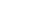is: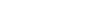where: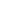= Windspeed vector

Aircraft flight instruments, however, don't compute true airspeed as a function of groundspeed and windspeed. They use impact and static pressures as well as a temperature input. True airspeed is equivalent airspeed that is corrected for pressure altitude
Pressure altitude
In aviation, pressure altitude is the indicated altitude when an altimeter is set to an agreed baseline pressure setting. The baseline pressure is 1013.25 hPa, equivalent to 1013.25 millibar, or 29.92 inches of mercury. This setting is equivalent to the air pressure at mean sea level in the...

and temperature (which define density). The result is the true physical speed of the aircraft plus or minus the wind component. True Airspeed is equal to calibrated airspeed and equivalent airspeed at standard sea level conditions.

The simplest way to compute true airspeed is using a function of Mach number
Mach number
Mach number is the speed of an object moving through air, or any other fluid substance, divided by the speed of sound as it is in that substance for its particular physical conditions, including those of temperature and pressure...

: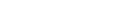Where: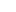= Speed of sound at standard sea level (661.4788 knots)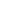= Mach number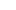= Temperature (kelvin
Kelvin
The kelvin is a unit of measurement for temperature. It is one of the seven base units in the International System of Units and is assigned the unit symbol K. The Kelvin scale is an absolute, thermodynamic temperature scale using as its null point absolute zero, the temperature at which all...

s)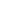= Standard sea level temperature (288.15 kelvins)

Or if Mach number is not known:Where:= Speed of sound at standard sea level (661.4788 knots)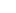= Impact pressure (inHg)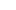= Static pressure (inHg)= Temperature (kelvins)= Standard sea level temperature (288.15 kelvin)

The above equation is only for Mach numbers less than 1.0.

True airspeed differs from the equivalent airspeed because the airspeed indicator is calibrated at SL, ISA conditions, where the air density is 1.225 kg/m³, whereas the air density in flight normally differs from this value.Thus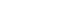Where: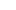is the air density at the flight condition.

The air density may be calculated from: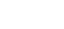Where :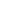is the air pressure at the flight condition,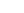is the air pressure at sea level = 1013.2 hPa,is the air temperature at the flight condition,is the air temperature at sea level, ISA
International Standard Atmosphere
The International Standard Atmosphere is an atmospheric model of how the pressure, temperature, density, and viscosity of the Earth's atmosphere change over a wide range of altitudes. It has been established to provide a common reference for temperature and pressure and consists of tables of...

= 288.15 K.

Source: Aerodynamics of a Compressible Fluid. Liepmann and Puckett 1947. Publishers John Wiley & Sons Inc.

## Groundspeed

Groundspeed is the speed of the aircraft relative to the ground rather than through the air, which can itself be moving.